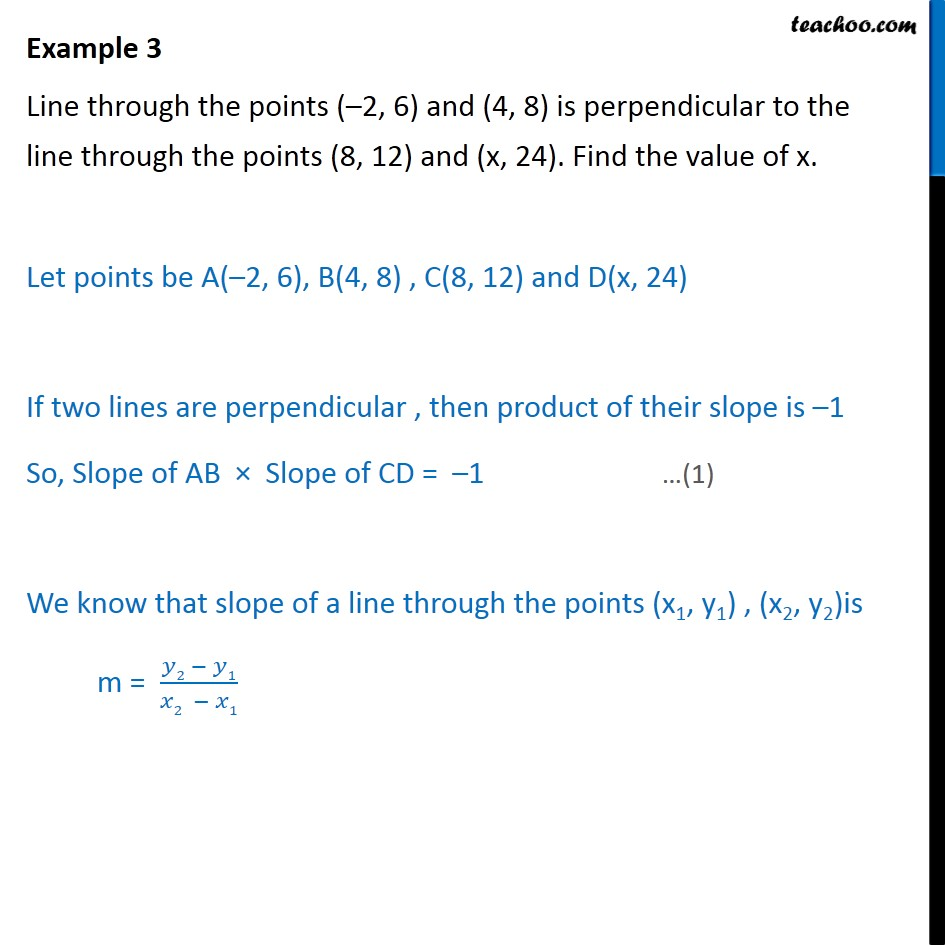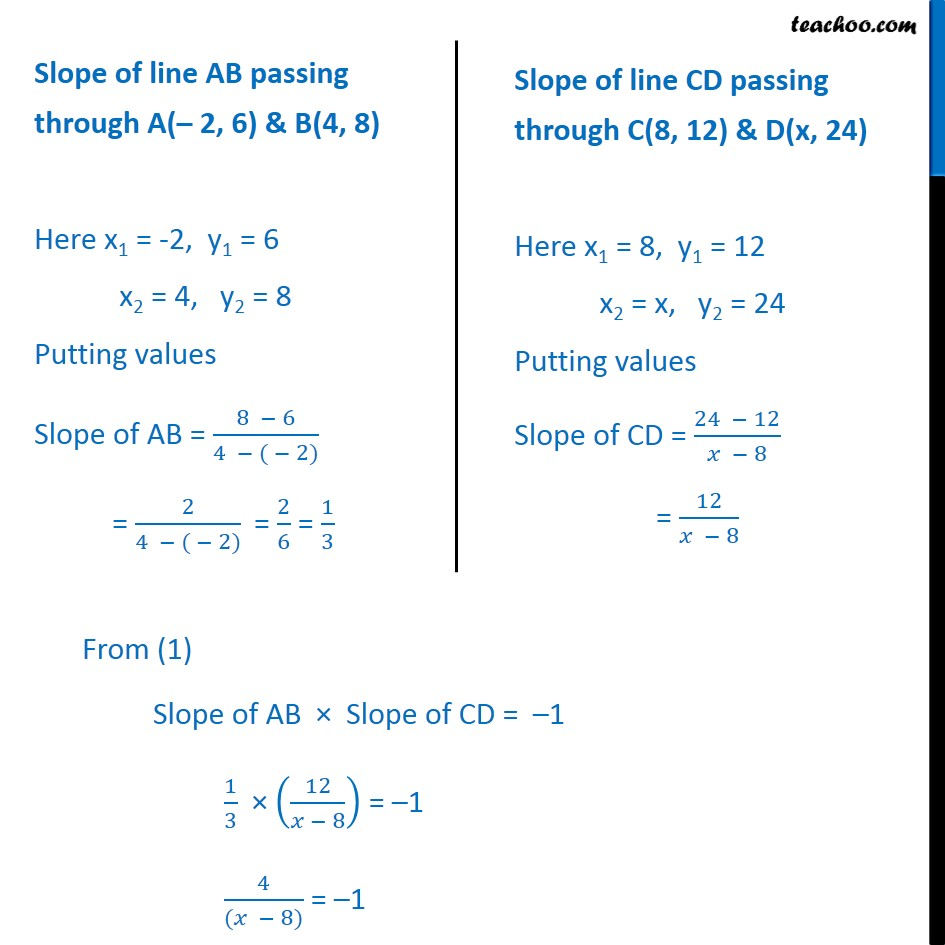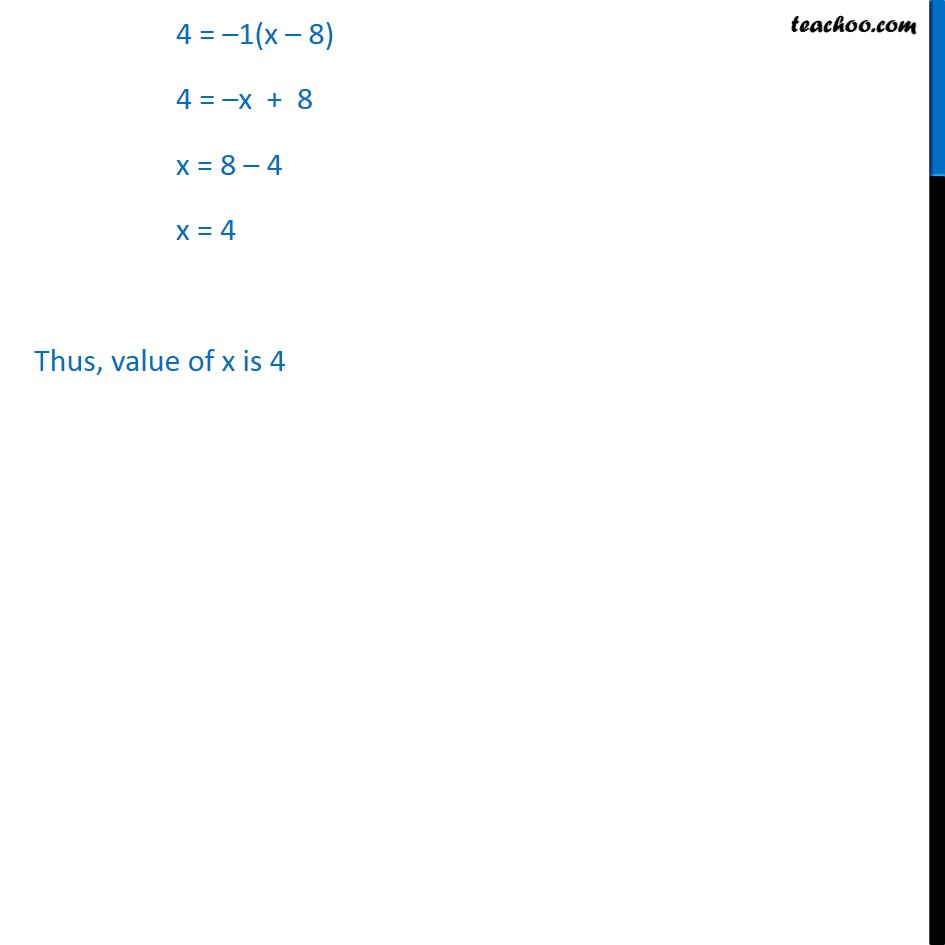1. Chapter 10 Class 11 Straight Lines
2. Concept wise
3. Slope - Prependicularity

Transcript

Example 3 Line through the points (–2, 6) and (4, 8) is perpendicular to the line through the points (8, 12) and (x, 24). Find the value of x. Let points be A(–2, 6), B(4, 8) , C(8, 12) and D(x, 24) If two lines are perpendicular , then product of their slope is –1 So, Slope of AB × Slope of CD = –1 We know that slope of a line through the points (x1, y1) , (x2, y2)is m = (𝑦2 − 𝑦1)/(𝑥2 − 𝑥1) 4 = –1(x – 8) 4 = –x + 8 x = 8 – 4 x = 4 Thus, value of x is 4

Slope - Prependicularity